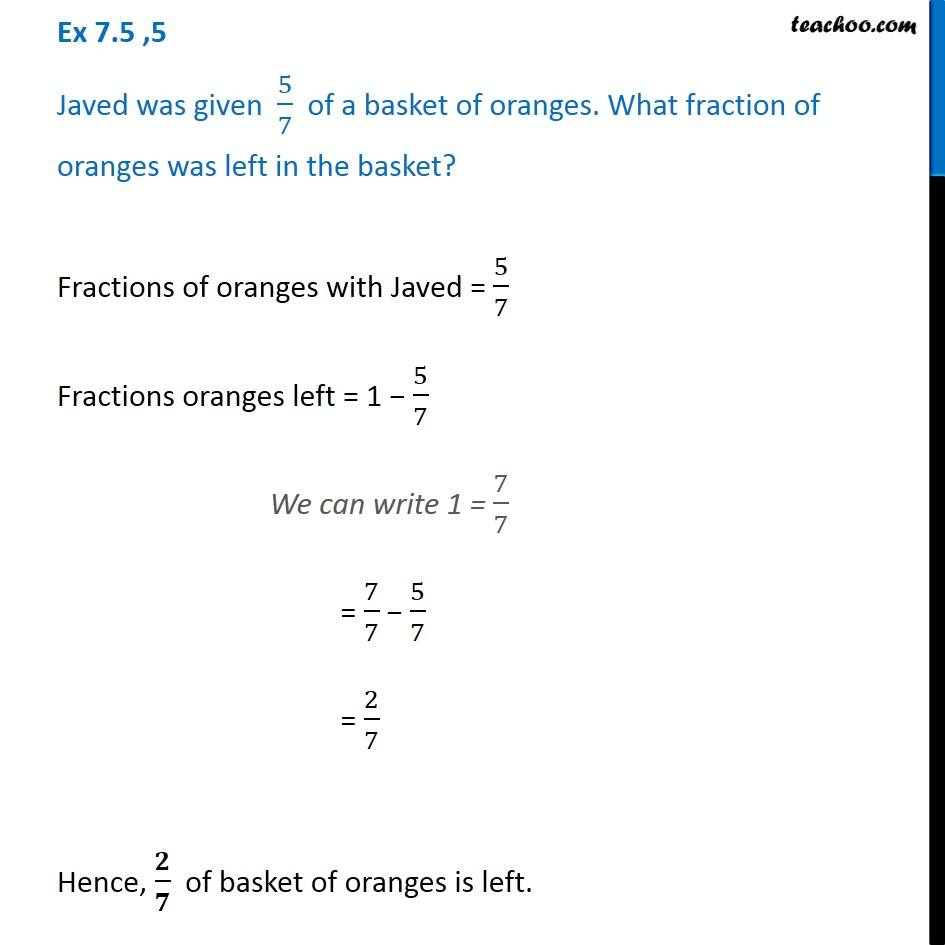1. Chapter 7 Class 6 Fractions
2. Serial order wise
3. Ex 7.5

Transcript

Ex 7.5 ,5 Javed was given 5/7 of a basket of oranges. What fraction of oranges was left in the basket? Fractions of oranges with Javed = 5/7 Fractions oranges left = 1 − 5/7 We can write 1 = 7/7 = 7/7 − 5/7 = 2/7 Hence, 𝟐/𝟕 of basket of oranges is left.

Ex 7.5

Chapter 7 Class 6 Fractions
Serial order wise